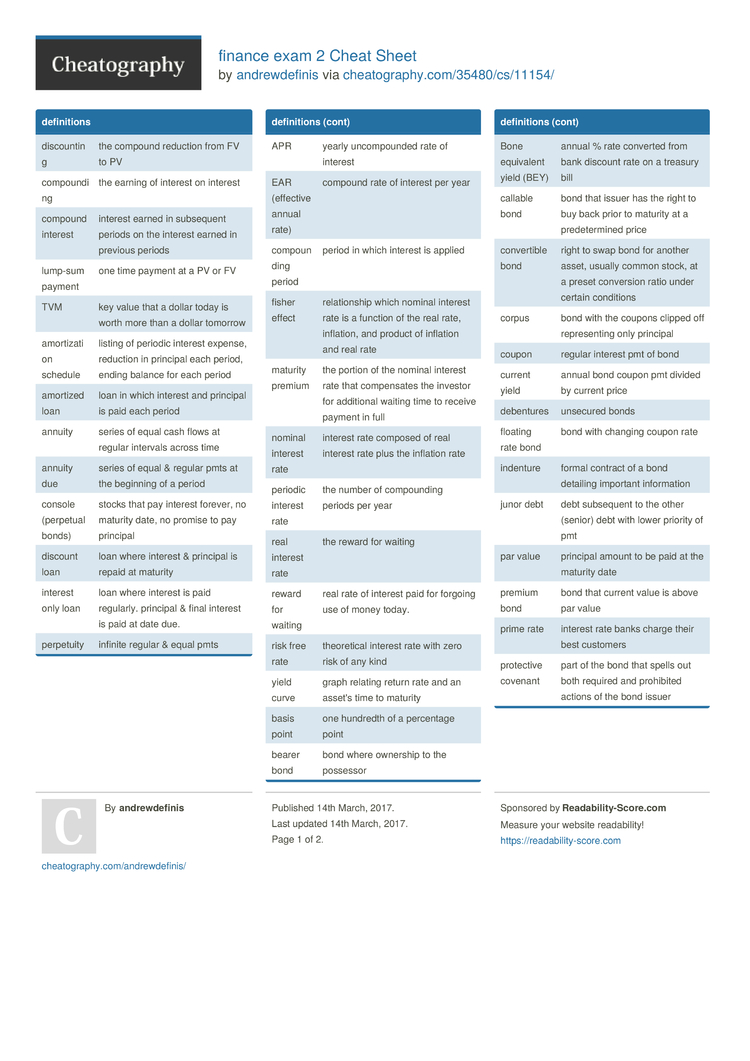# finance exam 2 Cheat Sheet by andrewdefinis

### defini­tions

 discou­nting the compound reduction from FV to PV compou­nding the earning of interest on interest compound interest interest earned in subsequent periods on the interest earned in previous periods lump-sum payment one time payment at a PV or FV TVM key value that a dollar today is worth more than a dollar tomorrow amorti­zation schedule listing of periodic interest expense, reduction in principal each period, ending balance for each period amortized loan loan in which interest and principal is paid each period annuity series of equal cash flows at regular intervals across time annuity due series of equal & regular pmts at the beginning of a period console (perpetual bonds) stocks that pay interest forever, no maturity date, no promise to pay principal discount loan loan where interest & principal is repaid at maturity interest only loan loan where interest is paid regularly. principal & final interest is paid at date due. perpetuity infinite regular & equal pmts APR yearly uncomp­ounded rate of interest EAR (effective annual rate) compound rate of interest per year compou­nding period period in which interest is applied fisher effect relati­onship which nominal interest rate is a function of the real rate, inflation, and product of inflation and real rate maturity premium the portion of the nominal interest rate that compen­sates the investor for additional waiting time to receive payment in full nominal interest rate interest rate composed of real interest rate plus the inflation rate periodic interest rate the number of compou­nding periods per year real interest rate the reward for waiting reward for waiting real rate of interest paid for forgoing use of money today. risk free rate theore­tical interest rate with zero risk of any kind yield curve graph relating return rate and an asset's time to maturity basis point one hundredth of a percentage point bearer bond bond where ownership to the possessor Bone equivalent yield (BEY) annual % rate converted from bank discount rate on a treasury bill callable bond bond that issuer has the right to buy back prior to maturity at a predet­ermined price conver­tible bond right to swap bond for another asset, usually common stock, at a preset conversion ratio under certain conditions corpus bond with the coupons clipped off repres­enting only principal coupon regular interest pmt of bond current yield annual bond coupon pmt divided by current price debentures unsecured bonds floating rate bond bond with changing coupon rate indenture formal contract of a bond detailing important inform­ation junor debt debt subsequent to the other (senior) debt with lower priority of pmt par value principal amount to be paid at the maturity date premium bond bond that current value is above par value prime rate interest rate banks charge their best customers protective covenant part of the bond that spells out both required and prohibited actions of the bond issuer putable bond bond holder has the right to sell the bond back to the issuer at a determined price prior to maturity sinking fund special fund for the retirement of debt on bonds STRIPS zero-c­oupon bonds made by separating the interest and principal on us. govt bonds treasury bill govt bond with a maturity less than one year treasury bond govt bond with maturity of more than ten years treasury note govt bond with a maturity between 2 and 10 years yield to call discount rate of return for a callable premium bond yield to maturity (YTM) return the holder receives if held on till maturity zero-c­oupon bond a bond that pays no coupons over its maturity

### formulas

 compou­nding interestFVn=PV­o(1­+r)^n (finding fv) PVo=FVo/ (1+r)^n (finding pv) n=ln(f­v/p­v)/­ln(1+r) (finding time) R= (FVo * PVo) ^ (1/n) -1 (finding rate) FVn=PMT [((1 + r)n - 1) / r] PVt =PMT(t+1) [(1 - 1/(1 + r)n) / r] PVt =PMT(t+1) / r Periodic Interest Rater = APR / m EAR =(1 + APR/m)m - 1 . r ≈r* + h r =r* + inf + dp + mp Bond Price (PB) =Coupon PMT [(1 - 1/(1 + r)n) / r] + Par / (1 + r)n Current Yield =Annual Coupon PMT / PB YTM =(Par/P­B)(1/n) – 1 YTM ≈(Coupon + (Par –PB)/n) / [.4 Par + .6 PB] Profit =ending value + distri­but­ion­s-o­riginal cost HPR =(profit or loss)/­ori­ginal cost Simple annual return =HPR/n EAR =(1 + HPR)(1/n) - 1 Variance (X) =[∑(Xi – Average)2] / (n – 1) = σ2 (Divisor of (n – 1) for sample and (n) for popula­tion) Standard Deviation =(σ2)(1/2) = σ Re =E (ri) = rf + βi [E(rm) - rf]

### excel2 Pages
//media.cheatography.com/storage/thumb/andrewdefinis_finance-exam-2.750.jpg

PDF (recommended)